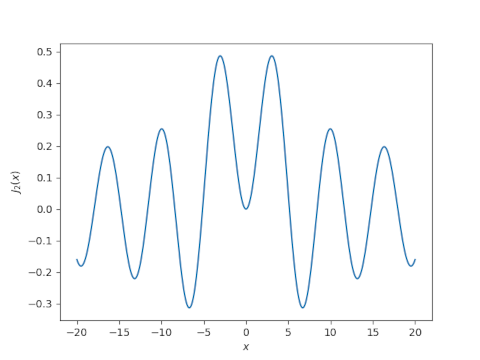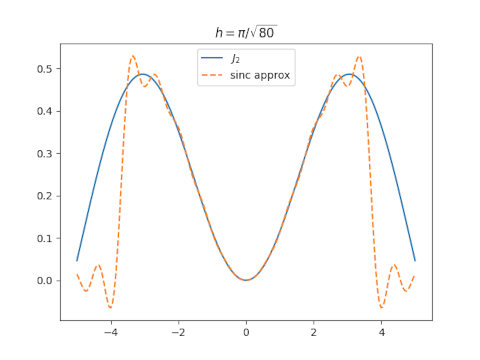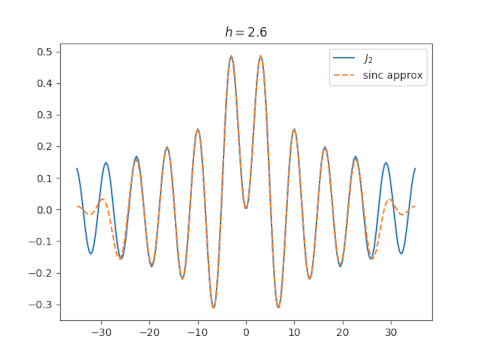# Sinc approximation to Bessel function

The Bessel functions Jn for even n look something like the sinc function. How well can you approximate the former by sums of the latter? To make things concrete, we’ll approximate J2. Here’s a plot of J2.And here’s a plot of sinc(x) = sin(πx)/πx.The sinc approximation for a function f(x) is given bySinc approximation can be remarkably accurate, nearly optimal in some sense.

The accuracy of the approximation increases as n gets larger and h gets smaller. We will fix n = 10. How should we pick h? The paper cited in this post suggests usingLet’s try that and see what happens.The approximation isn’t very good overall, though it’s excellent near 0.

Before making plots, I had a plausible argument for why the value of h suggested above might be optimal. I also had an argument for why a much larger value of h, something on the order of 8 might be optimal. Turns out both are wrong. You can get a good approximation over a larger range by choosing h around 2.6.